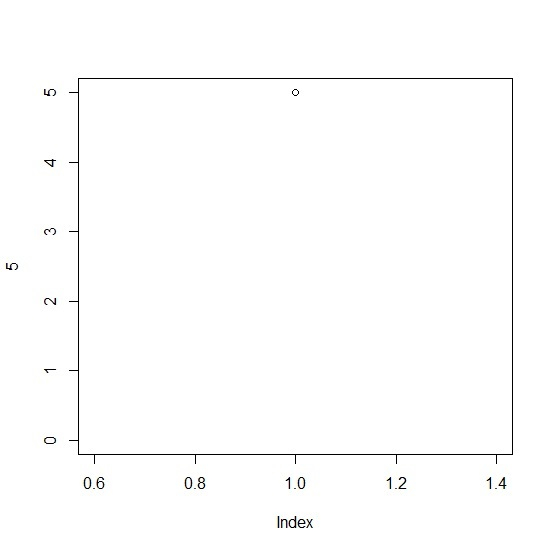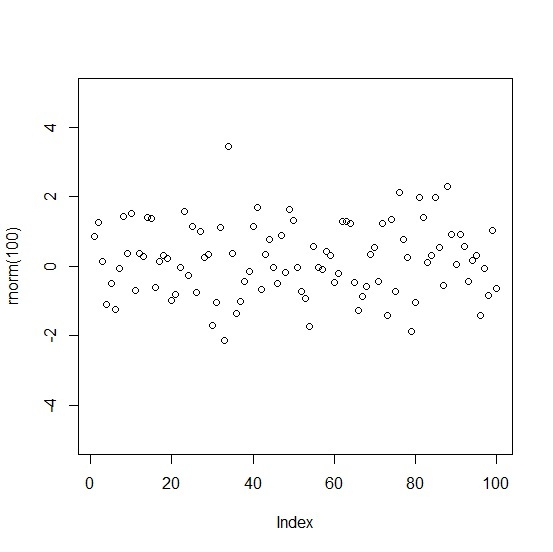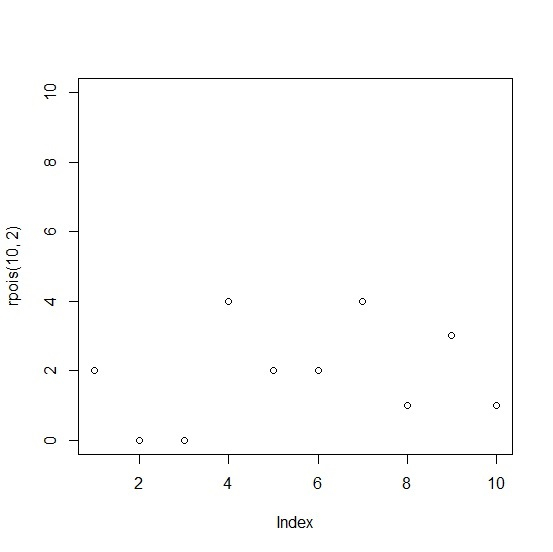# How to include a zero with tick in base R plot?

When we create a plot in base R the Y-axis values are generated automatically and mostly zero is now shown except in few cases that can’t be defined in particular but happens when there exists a zero in data. Therefore, if we want to include a zero with tick in base R plot then ylim argument can be used with the plot function.

## Example

plot(5,ylim=c(0,5))

## Output## Example

plot(rnorm(100),ylim=c(-5,5))

## Output## Example

plot(rpois(10,2),ylim=c(0,10))

## Output